Test: Probability- 1

# Test: Probability- 1

Test Description

## 10 Questions MCQ Test CSAT Preparation for UPSC CSE | Test: Probability- 1

Test: Probability- 1 for Quant 2022 is part of CSAT Preparation for UPSC CSE preparation. The Test: Probability- 1 questions and answers have been prepared according to the Quant exam syllabus.The Test: Probability- 1 MCQs are made for Quant 2022 Exam. Find important definitions, questions, notes, meanings, examples, exercises, MCQs and online tests for Test: Probability- 1 below.
Solutions of Test: Probability- 1 questions in English are available as part of our CSAT Preparation for UPSC CSE for Quant & Test: Probability- 1 solutions in Hindi for CSAT Preparation for UPSC CSE course. Download more important topics, notes, lectures and mock test series for Quant Exam by signing up for free. Attempt Test: Probability- 1 | 10 questions in 10 minutes | Mock test for Quant preparation | Free important questions MCQ to study CSAT Preparation for UPSC CSE for Quant Exam | Download free PDF with solutions
 1 Crore+ students have signed up on EduRev. Have you?
Test: Probability- 1 - Question 1

### A die is rolled twice. What is the probability of getting a sum equal to 9?

Detailed Solution for Test: Probability- 1 - Question 1

Total number of outcomes possible when a die is rolled = 6 (∵ any one face out of the 6 faces)

• Hence, total number of outcomes possible when a die is rolled twice, n(S) = 6 x 6 = 36

E = Getting a sum of 9 when the two dice fall = {(3,6), (4,5), (5,4), (6,3)}

• Hence, n(E) = 4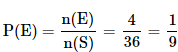Test: Probability- 1 - Question 2

### When tossing two coins once, what is the probability of heads on both the coins?

Detailed Solution for Test: Probability- 1 - Question 2

Total number of outcomes possible when a coin is tossed = 2 (∵ Head or Tail)

• Hence, total number of outcomes possible when two coins are tossed, n(S) = 2 x 2 = 4
(∵ Here, S = {HH,HT,TH,TT})

E = event of getting heads on both the coins = {HH}

• Hence, n(E) = 1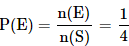Test: Probability- 1 - Question 3

### What is the probability of getting a number less than 4 when a die is rolled?

Detailed Solution for Test: Probability- 1 - Question 3
• Total number of outcomes possible when a die is rolled = 6
(∵ any one face out of the 6 faces) i.e., n(S) = 6
• E = Getting a number less than 4 = {1, 2, 3}
Hence, n(E) = 3Test: Probability- 1 - Question 4

One card is randomly drawn from a pack of 52 cards. What is the probability that the card drawn is a face card(Jack, Queen or King)

Detailed Solution for Test: Probability- 1 - Question 4
• Total number of cards, n(S) = 52
• Total number of face cards, n(E) = 12 (4 Jacks, 4 Queens, 4 Kings)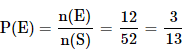Test: Probability- 1 - Question 5

A dice is thrown. What is the probability that the number shown in the dice is divisible by 3?

Detailed Solution for Test: Probability- 1 - Question 5
• Total number of outcomes possible when a die is rolled, n(S) = 6 (? 1 or 2 or 3 or 4 or 5 or 6)
• E = Event that the number shown in the dice is divisible by 3 = {3, 6}
Hence, n(E) = 2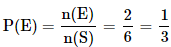Test: Probability- 1 - Question 6

John draws a card from a pack of cards. What is the probability that the card drawn is a card of black suit?

Detailed Solution for Test: Probability- 1 - Question 6

Total number of cards, n(S) = 52
Total number of black cards, n(E) = 26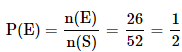Test: Probability- 1 - Question 7

Three coins are tossed. What is the probability of getting at most two tails?

Detailed Solution for Test: Probability- 1 - Question 7

Total number of outcomes possible when a coin is tossed = 2 (∵ Head or Tail)

• Hence, total number of outcomes possible when 3 coins are tossed, n(S) = 2 x 2 x 2 = 8
​(∵ S = {TTT, TTH, THT, HTT, THH, HTH, HHT, HHH})

E = event of getting at most two Tails = {TTH, THT, HTT, THH, HTH, HHT, HHH}

• Hence, n(E) = 7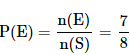Test: Probability- 1 - Question 8

A bag contains 4 black, 5 yellow and 6 green balls. Three balls are drawn at random from the bag. What is the probability that all of them are yellow?

Detailed Solution for Test: Probability- 1 - Question 8

Total number of balls = 4 + 5 + 6 = 15

Let S be the sample space.

• n(S) = Total number of ways of drawing 3 balls out of 15 = 15C3

Let E = Event of drawing 3 balls, all of them are yellow.

• n(E) = Number of ways of drawing 3 balls from the total 5 = 5C3
(∵ there are 5 yellow balls in the total balls)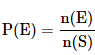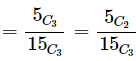[∵ nCr = nC(n-r). So 5C3 = 5C2. Applying this for the ease of calculation]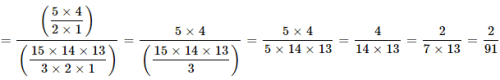Test: Probability- 1 - Question 9

A bag contains 2 yellow, 3 green and 2 blue balls. Two balls are drawn at random. What is the probability that none of the balls drawn is blue?

Detailed Solution for Test: Probability- 1 - Question 9

Total number of balls = 2 + 3 + 2 = 7

► Let S be the sample space.

• n(S) = Total number of ways of drawing 2 balls out of 7 = 7C2

► Let E = Event of drawing 2 balls, none of them is blue.

• n(E) = Number of ways of drawing 2 balls from the total 5 (= 7-2) balls = 5C2
(∵ There are two blue balls in the total 7 balls. Total number of non-blue balls = 7 - 2 = 5)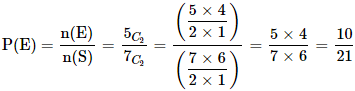Test: Probability- 1 - Question 10

There are 15 boys and 10 girls in a class. If three students are selected at random, what is the probability that 1 girl and 2 boys are selected?

Detailed Solution for Test: Probability- 1 - Question 10

Let S be the sample space.

• n(S) = Total number of ways of selecting 3 students from 25 students = 25C3

Let E = Event of selecting 1 girl and 2 boys

• n(E) = Number of ways of selecting 1 girl and 2 boys

15 boys and 10 girls are there in a class. We need to select 2 boys from 15 boys and 1 girl from 10 girls

Number of ways in which this can be done:
15C2 × 10C1
Hence n(E) = 15C2 × 10C1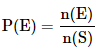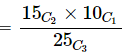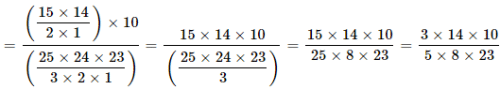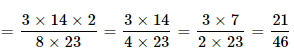## CSAT Preparation for UPSC CSE

72 videos|64 docs|92 tests
 Use Code STAYHOME200 and get INR 200 additional OFF Use Coupon Code
Information about Test: Probability- 1 Page
In this test you can find the Exam questions for Test: Probability- 1 solved & explained in the simplest way possible. Besides giving Questions and answers for Test: Probability- 1, EduRev gives you an ample number of Online tests for practice

## CSAT Preparation for UPSC CSE

72 videos|64 docs|92 tests